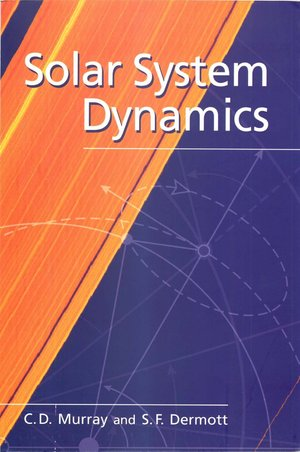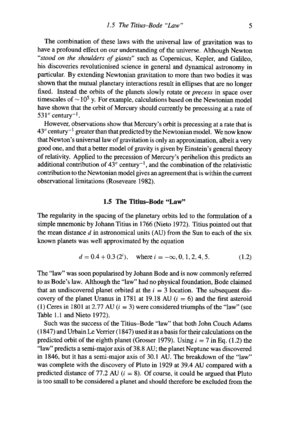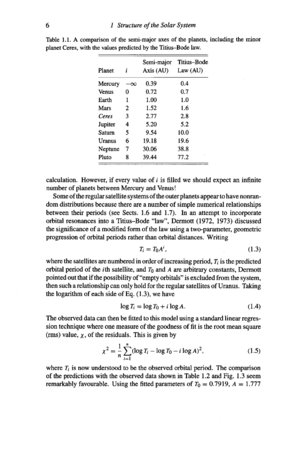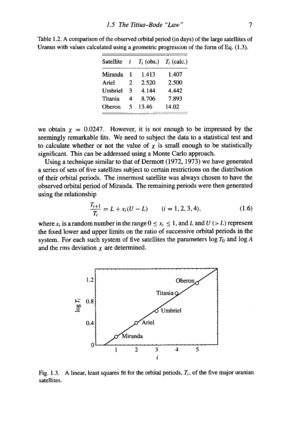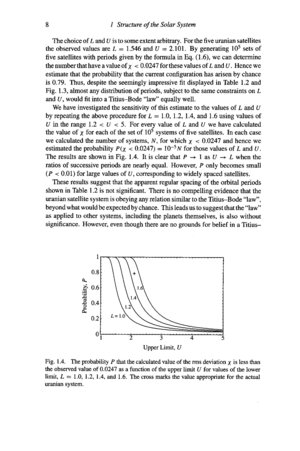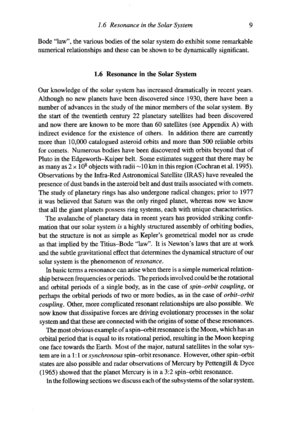M A T H 2 1 B
Mathematics Math21b Spring 2010
Linear Algebra and Differential Equations
Exhibit: Titius Brahe Law
 The following pages are from the book "Solar System Dynamics" by C.D. Murray and S.F. Dermott. In the homework you have done some datafitting to derive a Kepler law. Here is an other example of a data fitting problem in astronomy: the Titius-Brade Law: Brade's law was that the distance d from the sun in astronimical units from the sun to each of the six known planets is ```d = 0.4 + 0.3 wn, n=-infinity, 0,1,2,4,5 ``` The law predicted an other planet for n=3 and 6 etc. brode claimed a planet at n=3. After Uranus was found close to n=6, the law was considered a success and called Titius Bode law.